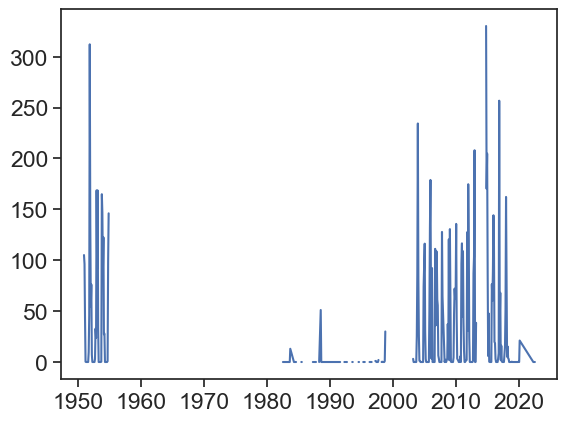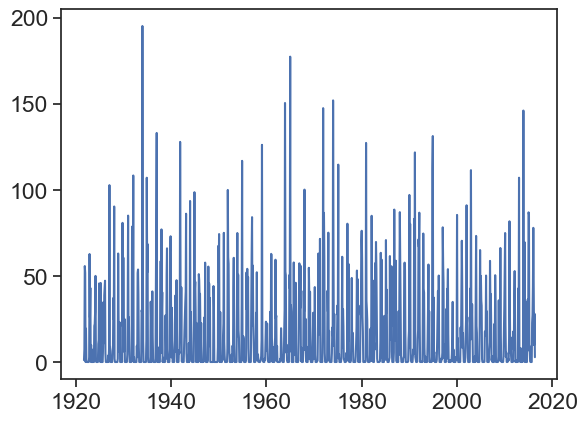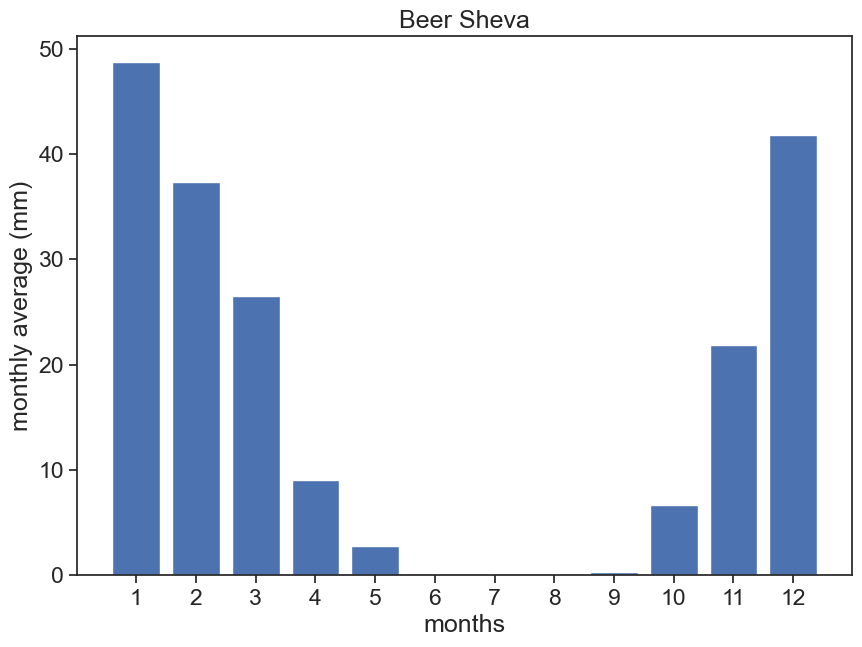Import relevant packages

import matplotlib.pyplot as plt
import numpy as np
import pandas as pd
from calendar import month_abbr
import seaborn as sns
sns.set(style="ticks", font_scale=1.5)
import urllib.request
from pandas.plotting import register_matplotlib_converters
register_matplotlib_converters()

## 6.1 intra-annual variability

Go to NOAA’s National Centers for Environmental Information (NCEI)
Climate Data Online: Dataset Discovery

Find station codes in this map. On the left, click on the little wrench (🔧) next to “Global Summary of the Month”, then click on “identify” on the panel that just opened, and click on a station (purple circle). You will see the station’s name, it’s ID, and the period of record. For example, for Ben-Gurion’s Airport in Israel:
BEN GURION, IS
STATION ID: ISM00040180
Period of Record: 1951-01-01 to 2020-03-01

def download_data(station_name, station_code):
url_daily = 'https://www.ncei.noaa.gov/data/global-historical-climatology-network-daily/access/'
url_monthly = 'https://www.ncei.noaa.gov/data/gsom/access/'
# download daily data - uncomment the next 2 lines to make this work
# urllib.request.urlretrieve(url_daily + station_code + '.csv',
#                            station_name + '_daily.csv')
urllib.request.urlretrieve(url_monthly + station_code + '.csv',
station_name + '_monthly.csv')

Now, choose any station with a period of record longer than 30 years, and download its data:

download_data('BEN_GURION', 'ISM00040180')

Load the data into a datafram, and before you continue with the analysis, plot the rainfall data, to see how it looks like.

download_data('BEN_GURION', 'ISM00040180')
# make 'DATE' the dataframe index
df['DATE'] = pd.to_datetime(df['DATE'])
df = df.set_index('DATE')
plt.plot(df['PRCP'])It doesn’t look great for Ben-Gurion airport, lots of missing data! You might need to choose another station… Download data for Beer Sheva, ID IS000051690.

download_data('BEER_SHEVA', 'IS000051690')
# make 'DATE' the dataframe index
df['DATE'] = pd.to_datetime(df['DATE'])
df = df.set_index('DATE')
plt.plot(df['PRCP'])That’s much better! We need to aggregate all data from each month, so we can calculate monthly averages. How to do that?

# choose only the precipitation column
df_month = df['PRCP']
# calculate monthly mean
monthly_mean = np.array([])  # empty array
month_numbers = np.arange(1,13)
month_names = [month_abbr[i] for i in month_numbers]

for m in month_numbers:      # cycle over months (1, 2, 3, etc)
this_month_all_indices = (df_month.index.month == m)       # indices in df_month belonging to month m
this_month_mean = df_month[this_month_all_indices].mean()  # this is the monthly mean
monthly_mean = np.append(monthly_mean, this_month_mean)    # append

Now it is time to create a new dataframe with the monthly means.

df_beersheva = pd.DataFrame({'monthly rainfall (mm)':monthly_mean,
'month names':month_names,
'month number':month_numbers
})
df_beersheva
monthly rainfall (mm) month names month number
0 48.743158 Jan 1
1 37.347368 Feb 2
2 26.551579 Mar 3
3 9.038947 Apr 4
4 2.735789 May 5
5 0.013830 Jun 6
6 0.000000 Jul 7
7 0.002128 Aug 8
8 0.271277 Sep 9
9 6.669474 Oct 10
10 21.850526 Nov 11
11 41.786316 Dec 12

Plot the data and see if it makes sense. Try to get a figure like this one.

fig, ax = plt.subplots(figsize=(10,7))
ax.bar(df_beersheva['month number'], df_beersheva['monthly rainfall (mm)'])
ax.set(xlabel="months",
ylabel="monthly average (mm)",
title="Beer Sheva",
xticks=df_beersheva['month number'],
xticklabels=df_beersheva['month names']);
plt.savefig("hydrology_figures/beersheva_monthly_average.png")Let’s calculate now the Walsh and Lawler Seasonality Index (leddris (2010), Walsh and Lawler (1981)) Write a function that receives a dataframe like the one we have just created, and returns the seasonality index.

$$R=$$ mean annual precipitation
$$m_i$$ precipitation mean for month $$i$$

$SI = \displaystyle \frac{1}{R} \sum_{n=1}^{n=12} \left| m_i - \frac{R}{12} \right|$

SI Precipitation Regime
<0.19 Precipitation spread throughout the year
0.20-0.39 Precipitation spread throughout the year, but with a definite wetter season
0.40-0.59 Rather seasonal with a short dry season
0.60-0.79 Seasonal
0.80-0.99 Marked seasonal with a long dry season
1.00-1.19 Most precipitation in < 3 months
def walsh_index(df):
mi = df["monthly rainfall (mm)"]
R = df["monthly rainfall (mm)"].sum()
SI = np.sum(np.abs(mi - R/12)) / R
return SI
beersheva_SI = walsh_index(df_beersheva)
print(f"Beer Sheva, SI = {beersheva_SI:.2f}")
Beer Sheva, SI = 0.97